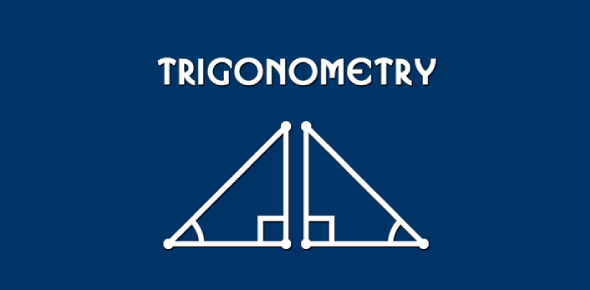# Trivia Quiz: Trigonometry Practice Test!

15 Questions | Total Attempts: 4757Settings.

Related Topics
• 1.
Value of , for = 1 Where 0° < < 90° is:
• A.

30°

• B.

60°

• C.

45°

• D.

135°

• 2.
Value of sec2 26° – cot2 64° is:
• A.

0

• B.

1

• C.

-1

• D.

2

• 3.
Product tan 1° tan 2° tan 3°………….. tan 89° is:
• A.

1

• B.

0

• C.

-1

• D.

90

• 4.
is equal to:
• A.
• B.
• C.
• D.
• 5.
• A.
• B.
• C.
• D.
• 6.
Maximum value of  is:
• A.

-1

• B.

2

• C.

1

• D.
• 7.
If  then value of
• A.

30°

• B.

60°

• C.

90°

• D.

120°

• 8.
If sin (A + B) = 1 = cos (A – B) then
• A.

A = B = 90°

• B.

A = B = 0°

• C.

A = B = 45°

• D.

A = 2B

• 9.
The value of 5 cos 00 + sin 900 is:
• A.

5

• B.

6

• C.

7

• D.

8

• 10.
How does the value of cos x varies when x increases from 00 to 900:
• A.

Decreases

• B.

Increases

• C.

Increases and then decreases

• D.

Decreases and then increases

• 11.
If sin A = sin A, then the value of BC from the figure is:
• A.

12

• B.

10

• C.

8

• D.

6

• 12.
Find the value of sin2 540 - cos2 360.
• A.

-1

• B.

1

• C.

2

• D.

0

• 13.
Cos 450 + tan 450 - sin 450 is:
• A.

1

• B.

2

• C.

-2

• D.

-1

• 14.
If tan A = 1, find the value of sin A:
• A.
• B.
• C.

-1

• D.

1

• 15.
If  then what is the value of sec B; given A + B = 1:
• A.

-1

• B.

2

• C.

1

• D.

0